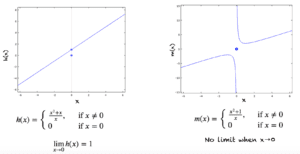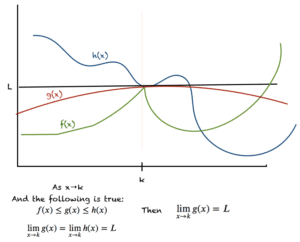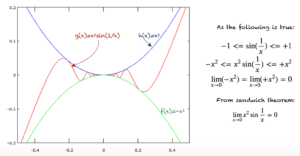# Case for Functions with a Discontinuity

Suppose we have a function h(x), which is defined for all real numbers:

h(x) = (x^2+x)/x, if x≠0

h(x) = 0, if x=0

The function g(x), has a discontinuity at x=0, as shown in the figure below. When evaluating lim(x→0)h(x), we have to see what happens to h(x) when x is close to 0 (and not when x=0). As we approach x=0 from either side, h(x) approaches 1, and hence lim(x→0)h(x)=1.

The function m(x) shown in the figure below is another interesting case. This function is also defined for all real numbers but the limit does not exist when x→0.Evaluating Limits when there is a Discontinuity

## The Sandwich Theorem

This theorem is also called the Squeeze theorem or the Pinching theorem. It states that when the following are true:

1. x is close to k
2. f(x) <= g(x) <= h(x)
3. lim(x→k)f(x) = lim(x→k)h(x) = L

then the limit of g(x) as x approaches k is given by:

lim(x→k)g(x) = LThe Sandwich Theorem

This theorem is illustrated in the figure below:

Using this theorem we can evaluate the limits of many complex functions. A well known example involves the sine function:

lim(x→0)x^2sin(1/x)Computing Limits Using Sandwich Theorem

We know that the sin(x) always alternates between -1 and +1. Using this fact, we can solve this limit as shown below: